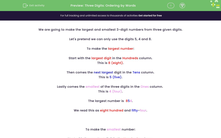# Three Digits: Ordering by Words

In this worksheet, students use number words to identify the smallest and largest options of three given digits.Key stage:  KS 2

Curriculum topic:   Number: Number and Place Value

Curriculum subtopic:   Order/Compare Numbers to 1000

Difficulty level:#### Worksheet Overview

We are going to make the largest and smallest 3-digit numbers from three given digits.

Let's pretend we can only use the digits 5, 4 and 8.

To make the largest number:

This is 8 (eight).

Then comes the next largest digit in the Tens column.

This is 5 (five).

Lastly comes the smallest of the three digits in the Ones column.

This is 4 (four).

The largest number is  854.

We read this as eight hundred and fifty-four.

To make the smallest number:

This is 4 (four).

Then comes the next largest digit in the Tens column.

This is 5 (five).

Lastly comes the largest of the three digits in the Units or Ones column.

This is 8 (eight).

The smallest number is  458.

We read this as four hundred and fifty-eight.

Example

Use each of the digits:

686

to form the smallest and largest 3-digit numbers that you can make.

6 is the smallest digit.

6 is the "middle" digit even though it is the same as the smallest here.

8 is the largest digit.

The smallest number is 668.

We read this as six hundred and sixty-eight.

Largest number is 866.

We read this as eight hundred and sixty-six.

### What is EdPlace?

We're your National Curriculum aligned online education content provider helping each child succeed in English, maths and science from year 1 to GCSE. With an EdPlace account you’ll be able to track and measure progress, helping each child achieve their best. We build confidence and attainment by personalising each child’s learning at a level that suits them.

Get started